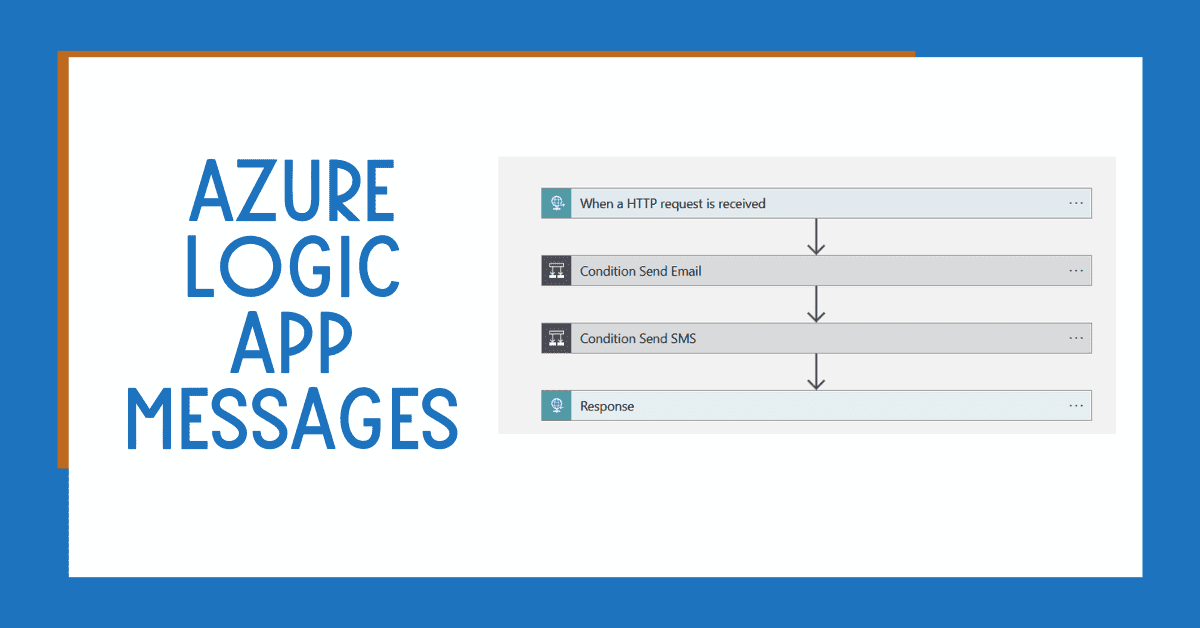Actionable Data Analytics

It’s important to monitor table size in Azure Synapse Analytics Dedicated SQL Pools. In this post, you’ll learn the queries you need to monitor table size. This information will help you understand if the table type and distribution are still suitable for your table (example: Replicated, Round-robin, Hash-distributed).

## Why should I monitor table size in Azure Synapse Analytics SQL Dedicated Pools?

To begin, the design of the tables in your Azure Synapse Analytics Dedicated SQL Pools plays a critical role in improving the performance of the queries in your database. Having replicated tables for your small lookup information or choosing the right distribution key for your table helps you avoid performance issues.

Monitoring table size is not a new practice. It has always been important to help plan data growth or understand savings when trying to archive information.

Additionally, the added complexity in Azure Synapse Analytics Dedicated Pools is that the information is stored across multiple logical distributions. Each distribution could be storing a different number of rows for the same table (aka distributed key). Therefore, when trying to get the size of a table, you need to add the size of all the distributions.

The queries below have been copied from Microsoft documentation

## Creating a View to Ease Analysis

The first step is to create a view to ease analysis. The following query will create the table vTableSizes in your database.

```				```
CREATE VIEW dbo.vTableSizes
AS
WITH base
AS
(
SELECT
GETDATE()                                                             AS  [execution_time]
, DB_NAME()                                                            AS  [database_name]
, s.name                                                               AS  [schema_name]
, t.name                                                               AS  [table_name]
, QUOTENAME(s.name)+'.'+QUOTENAME(t.name)                              AS  [two_part_name]
, nt.[name]                                                            AS  [node_table_name]
, ROW_NUMBER() OVER(PARTITION BY nt.[name] ORDER BY (SELECT NULL))     AS  [node_table_name_seq]
, tp.[distribution_policy_desc]                                        AS  [distribution_policy_name]
, c.[name]                                                             AS  [distribution_column]
, nt.[distribution_id]                                                 AS  [distribution_id]
, i.[type]                                                             AS  [index_type]
, i.[type_desc]                                                        AS  [index_type_desc]
, nt.[pdw_node_id]                                                     AS  [pdw_node_id]
, pn.[type]                                                            AS  [pdw_node_type]
, pn.[name]                                                            AS  [pdw_node_name]
, di.name                                                              AS  [dist_name]
, di.position                                                          AS  [dist_position]
, nps.[partition_number]                                               AS  [partition_nmbr]
, nps.[reserved_page_count]                                            AS  [reserved_space_page_count]
, nps.[reserved_page_count] - nps.[used_page_count]                    AS  [unused_space_page_count]
, nps.[in_row_data_page_count]
+ nps.[row_overflow_used_page_count]
+ nps.[lob_used_page_count]                                        AS  [data_space_page_count]
, nps.[reserved_page_count]
- (nps.[reserved_page_count] - nps.[used_page_count])
- ([in_row_data_page_count]
+ [row_overflow_used_page_count]+[lob_used_page_count])       AS  [index_space_page_count]
, nps.[row_count]                                                      AS  [row_count]
from
sys.schemas s
INNER JOIN sys.tables t
ON s.[schema_id] = t.[schema_id]
INNER JOIN sys.indexes i
ON  t.[object_id] = i.[object_id]
AND i.[index_id] <= 1
INNER JOIN sys.pdw_table_distribution_properties tp
ON t.[object_id] = tp.[object_id]
INNER JOIN sys.pdw_table_mappings tm
ON t.[object_id] = tm.[object_id]
INNER JOIN sys.pdw_nodes_tables nt
ON tm.[physical_name] = nt.[name]
INNER JOIN sys.dm_pdw_nodes pn
ON  nt.[pdw_node_id] = pn.[pdw_node_id]
INNER JOIN sys.pdw_distributions di
ON  nt.[distribution_id] = di.[distribution_id]
INNER JOIN sys.dm_pdw_nodes_db_partition_stats nps
ON nt.[object_id] = nps.[object_id]
AND nt.[pdw_node_id] = nps.[pdw_node_id]
AND nt.[distribution_id] = nps.[distribution_id]
LEFT OUTER JOIN (select * from sys.pdw_column_distribution_properties where distribution_ordinal = 1) cdp
ON t.[object_id] = cdp.[object_id]
LEFT OUTER JOIN sys.columns c
ON cdp.[object_id] = c.[object_id]
AND cdp.[column_id] = c.[column_id]
WHERE pn.[type] = 'COMPUTE'
)
, size
AS
(
SELECT
[execution_time]
,  [database_name]
,  [schema_name]
,  [table_name]
,  [two_part_name]
,  [node_table_name]
,  [node_table_name_seq]
,  [distribution_policy_name]
,  [distribution_column]
,  [distribution_id]
,  [index_type]
,  [index_type_desc]
,  [pdw_node_id]
,  [pdw_node_type]
,  [pdw_node_name]
,  [dist_name]
,  [dist_position]
,  [partition_nmbr]
,  [reserved_space_page_count]
,  [unused_space_page_count]
,  [data_space_page_count]
,  [index_space_page_count]
,  [row_count]
,  ([reserved_space_page_count] * 8.0)                                 AS [reserved_space_KB]
,  ([reserved_space_page_count] * 8.0)/1000                            AS [reserved_space_MB]
,  ([reserved_space_page_count] * 8.0)/1000000                         AS [reserved_space_GB]
,  ([reserved_space_page_count] * 8.0)/1000000000                      AS [reserved_space_TB]
,  ([unused_space_page_count]   * 8.0)                                 AS [unused_space_KB]
,  ([unused_space_page_count]   * 8.0)/1000                            AS [unused_space_MB]
,  ([unused_space_page_count]   * 8.0)/1000000                         AS [unused_space_GB]
,  ([unused_space_page_count]   * 8.0)/1000000000                      AS [unused_space_TB]
,  ([data_space_page_count]     * 8.0)                                 AS [data_space_KB]
,  ([data_space_page_count]     * 8.0)/1000                            AS [data_space_MB]
,  ([data_space_page_count]     * 8.0)/1000000                         AS [data_space_GB]
,  ([data_space_page_count]     * 8.0)/1000000000                      AS [data_space_TB]
,  ([index_space_page_count]  * 8.0)                                   AS [index_space_KB]
,  ([index_space_page_count]  * 8.0)/1000                              AS [index_space_MB]
,  ([index_space_page_count]  * 8.0)/1000000                           AS [index_space_GB]
,  ([index_space_page_count]  * 8.0)/1000000000                        AS [index_space_TB]
FROM base
)
SELECT * FROM size;
```
```

## Table sizes analysis in your Azure Synapse Analytics

Now, you can use the following queries to analyze table size.

### Azure Synapse Analytics Table Space Summary

Query for displaying the summary:

```				```
-- Table space summary
-- This query returns the rows and space by table.
-- It allows you to see which tables are your largest tables and whether they're round-robin, replicated,
-- or hash -distributed. For hash-distributed tables, the query shows the distribution column.
SELECT
database_name
,    schema_name
,    table_name
,    distribution_policy_name
,      distribution_column
,    index_type_desc
,    COUNT(distinct partition_nmbr) as nbr_partitions
,    SUM(row_count)                 as table_row_count
,    SUM(reserved_space_GB)         as table_reserved_space_GB
,    SUM(data_space_GB)             as table_data_space_GB
,    SUM(index_space_GB)            as table_index_space_GB
,    SUM(unused_space_GB)           as table_unused_space_GB
FROM
dbo.vTableSizes
GROUP BY
database_name
,    schema_name
,    table_name
,    distribution_policy_name
,      distribution_column
,    index_type_desc
ORDER BY
table_reserved_space_GB desc
;
```
```

The results display a summary of table sizes and distribution types.

### Azure Synapse Analytics Table Size by Distribution Type Query

Table size by distribution type query:

```				```
-- Table space by distribution type
SELECT
distribution_policy_name
,    SUM(row_count)                as table_type_row_count
,    SUM(reserved_space_GB)        as table_type_reserved_space_GB
,    SUM(data_space_GB)            as table_type_data_space_GB
,    SUM(index_space_GB)           as table_type_index_space_GB
,    SUM(unused_space_GB)          as table_type_unused_space_GB
FROM dbo.vTableSizes
GROUP BY distribution_policy_name
;
```
```

Results:

### Azure Synapse Analytics Table Size by Index Type

Table size by index type query:

```				```
-- Table space by index type
SELECT
index_type_desc
,    SUM(row_count)                as table_type_row_count
,    SUM(reserved_space_GB)        as table_type_reserved_space_GB
,    SUM(data_space_GB)            as table_type_data_space_GB
,    SUM(index_space_GB)           as table_type_index_space_GB
,    SUM(unused_space_GB)          as table_type_unused_space_GB
FROM dbo.vTableSizes
GROUP BY index_type_desc
;
```
```

Results:

### Azure Synapse Analytics Distribution Size Summary

Distribution size summary query:

```				```
-- Distribution space summary
SELECT
distribution_id
,    SUM(row_count)                as total_node_distribution_row_count
,    SUM(reserved_space_MB)        as total_node_distribution_reserved_space_MB
,    SUM(data_space_MB)            as total_node_distribution_data_space_MB
,    SUM(index_space_MB)           as total_node_distribution_index_space_MB
,    SUM(unused_space_MB)          as total_node_distribution_unused_space_MB
FROM dbo.vTableSizes
GROUP BY     distribution_id
ORDER BY    distribution_id
;
```
```

Results:

## Summary

In summary, correct table design is critical to maintain and improve the performance of your queries in Azure Synapse Analytics SQL Dedicated Pools. The queries mentioned in this post will help you identify how data is distributed across different tables. Then, you can choose an optimal table type for your data assets.

### WHAT’S NEXT?

In upcoming blog posts, we’ll continue to explore some of the features within Azure Services.